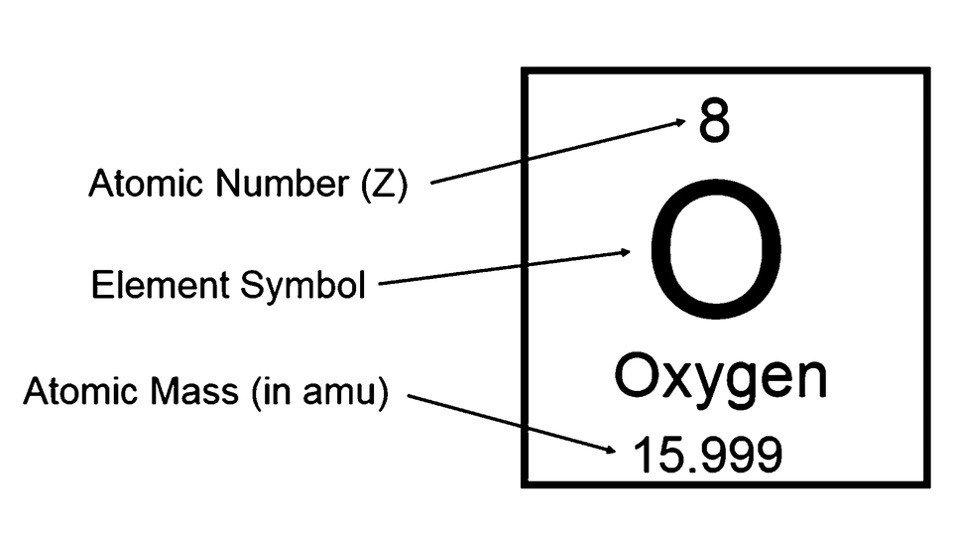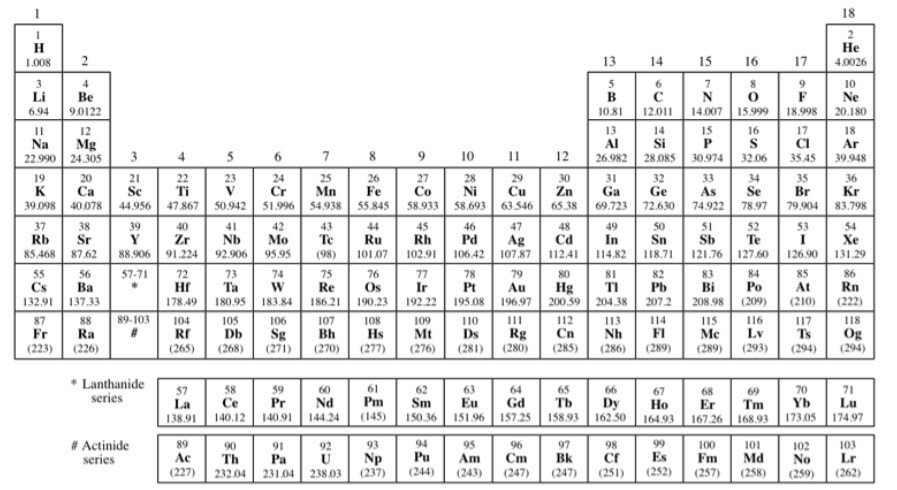Research Tweet

# What is Atomic Mass?

A property that is thoroughly related to an atom’s mass number is its atomic mass. T

he atomic mass of a single atom is basically defined as its total mass and is naturally expressed in its atomic mass units or is also called as amu.

In other words, an atom of carbon has six neutrons, carbon-12, with an atomic mass of 12 amu.

In general, however, an atom’s atomic mass is very close to its given mass number but will have some deviation in its decimal places.

Since an element’s isotopes have different atomic masses, researchers may also govern the relative atomic mass which is sometimes called as the atomic weight of an element.

The relative atomic mass is defined as an average of the atomic masses of all the different isotopes present in a sample.

Celestial objects such as asteroids or meteors may have a very different isotope abundance.

## How to Find Atomic Mass?

The figure given below explains how to find atomic mass and atomic number from a given element;### Atomic Mass Definition

Atomic mass is defined as the total mass of an atom. For example; the mass of 12C (carbon twelve) is inscribed as 12 amu.

All the masses of the elements are determined with 12C.

#### Periodic Table With Atomic Mass

The figure (periodic table with atomic mass) below shows the atomic mass of some of the frequently used elements;##### What is Atomic Number?

The atomic number of an element describes the element’s identity and indicates the number of protons present in the nucleus of that particular atom.

For instance, the element hydrogen always has one proton present in its nucleus. The element helium always has two protons present in its nucleus.

Similarly, from the above figure element molybdenum have 42 protons present in its nucleus.

###### Atomic Mass and Isotopes

Atoms of the same element can, have different numbers of neutrons present in their nucleus.

For any assumed isotope, the summation of the numbers of protons and neutrons in the nucleus is known as the mass number.

This is because each proton and each neutron present in the nucleus weigh one atomic mass unit (amu).

By totaling together the number of protons and neutrons and multiplying it by 1 amu, we can calculate the mass of the given atom.

The word ‘isotope’ originates from the Greek word ‘isos’ which means ‘same’ and ‘topes’ means ‘place’.

Thus the name isotopes to given to elements having the same number of protons because these elements can occupy a similar place on the periodic table while being different in their subatomic construction.

For instance, Carbon exists as two main isotopes, 12C, and 14C carbon atom which has the same number of protons and electrons, 12C has 6 neutrons, and 14C has 8 neutrons and is a radioactive isotope.

###### Isotopes Types

• Few isotopes do have unstable atomic nuclei that undergo radioactive decay. Particularly these isotopes are radioactive and are, consequently, known as radioisotopes or radionuclides.

Examples for the same include carbon-14, chlorine-36, uranium-235, and uranium-238, etc.

• Some isotopes are identified to have tremendously long half-lives (hundreds of millions of years of life). Such isotopes are generally stated as stable nuclides or stable isotopes.

Some examples for the same include carbon-12, carbon-13, oxygen-17, and oxygen-18, etc.

• Primordial nuclides are defined as the nuclides that have existed since the formation of this solar system.

Out of 339 naturally occurring isotopes present on the Earth, a whole of 286 isotopes are recognized to be primordial isotopes.

###### Why Atomic Mass is Useful?

The atomic mass is beneficial in chemistry when it is connected with the mole concept.

The atomic mass of an element that is measured in amu, is similar as the mass present in grams of one mole of an element.

For instance, the atomic mass of iron is 55.847 amu and one mole of iron atoms also weigh 55.847 grams.

Share

###### Similar Post: